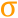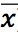# Standard Deviation

 Block Group: Statistical Functions Icon:The Standard Deviation block calculates standard deviation based on a list of arguments. The standard deviation is a measure of how widely values are dispersed from the mean.

The Standard Deviation block uses the following expression:

In this expression, x is the sample value,is the mean of all x values, and n is the sample size.

#### Note

If none of the input n values is a number, the block outputs NaN.

For information on standard deviation, see Standard deviation on Wikipedia.

For information on using dataflow blocks, see Dataflow.

For accepted and excluded arguments of Statistical Functions blocks, see Statistical Functions.

## Input/Output Property

The following property of the Standard Deviation block can take input and give output.

• input n (number)

input n defines one of the numbers in the sample.

## Output Property

The following property of the Standard Deviation block can give output but cannot take input.

• output (number)

output returns the standard deviation of the input values.

## Example

The following image shows an example of the Standard Deviation block.